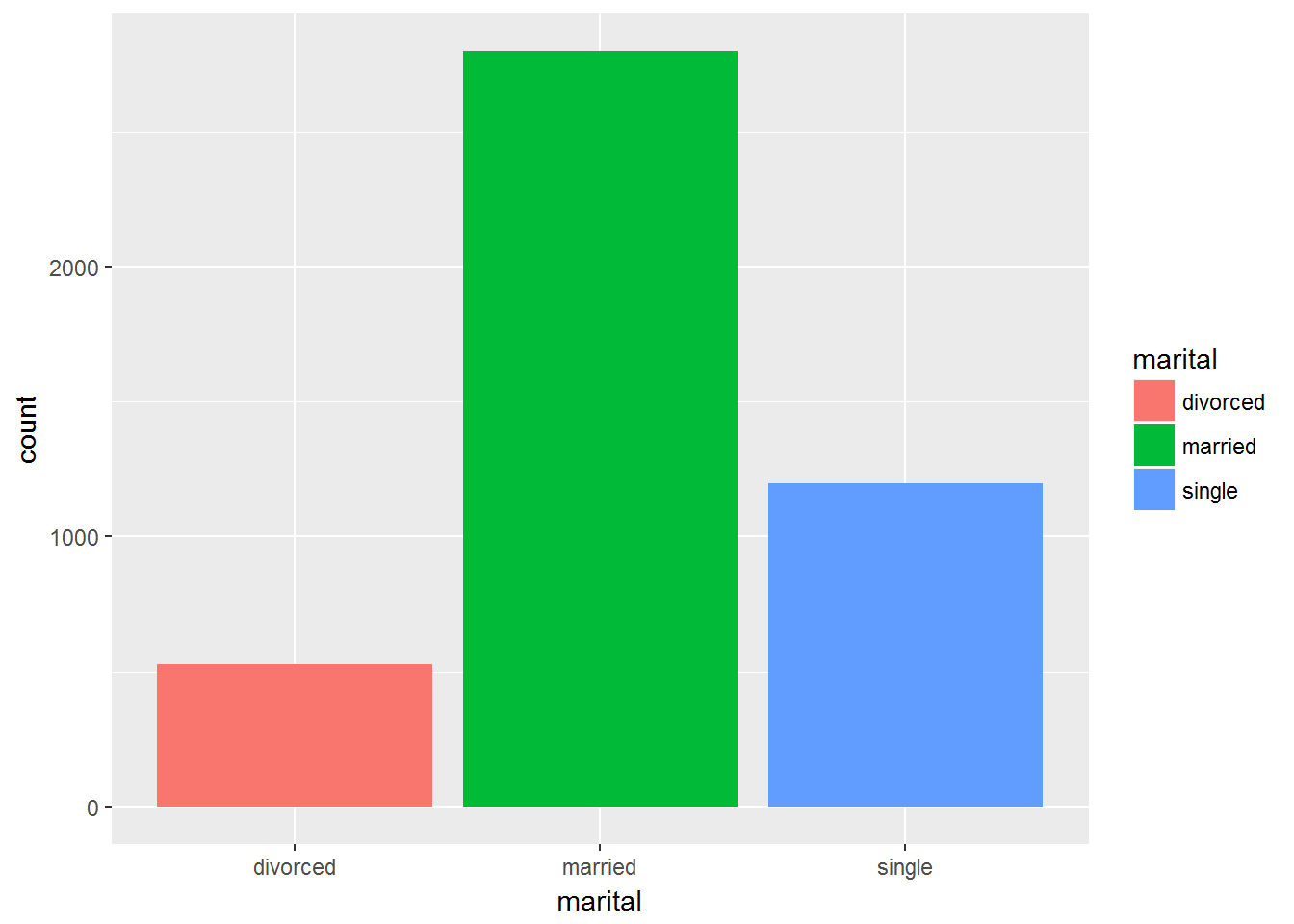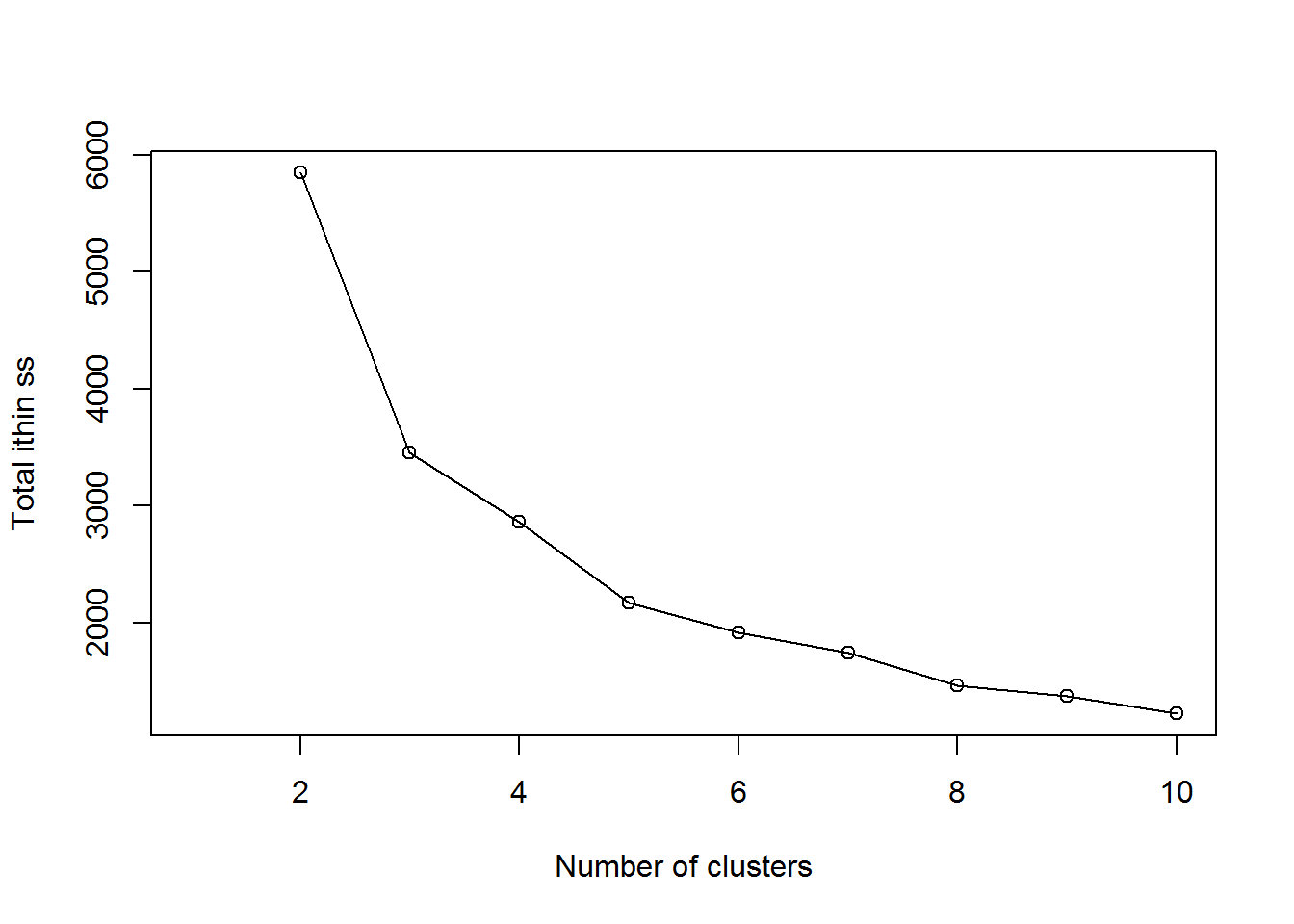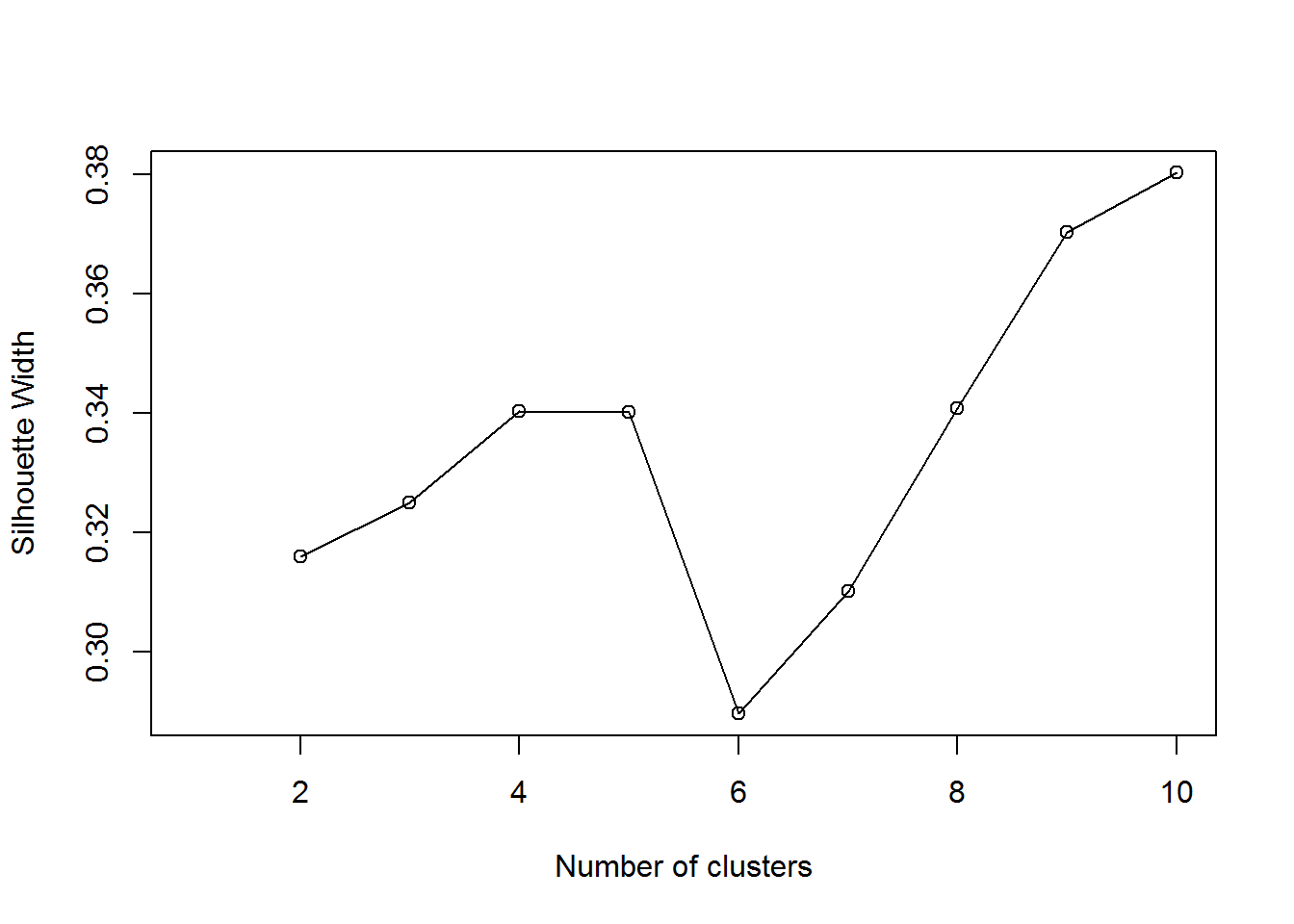### Storytelling with dataPhoto by rawpixel on Unsplash
Κάποια πολύ καλά tutorials είναι τα παρακάτω:
• Η σελίδα του twooctobers.com "8 Data Storytelling Concepts (with Examples!)" όπου περιγράφει κάποιες απαραίτητες, γενικές έννοιες και δίνονται χρήσιμες συμβουλές. Μερικές συνοψίζονται στην παρακάτω εικόνα.
Μερικοί ακόμη σύνδεσμοι:
• Για showcases συμβουλευτείτε τα:

Bank marketing

## Introduction

This is a simple example of several data analytics methods. The example is based on bank marketing dataset which is publicly available from UCI https://archive.ics.uci.edu/ml/datasets/bank+marketing. To be more precise, we’ll be using the csv file bank.csv located in the zip file https://archive.ics.uci.edu/ml/machine-learning-databases/00222/bank.zip.
The dataset contains the results of a Spain’s bank marketing campaing that tried to persuade clients to open a term deposit account.
For the scope of this example let’s suppose that we are assigned the task of:
• performing a simple segmentation of the clients
• detecting the most important factors that influence a clients’ decission
Those who attended the lecture at 2018’s summer scholl of Business Mathematics postgraduate program might remember that excel was used to create basic descriptve plots of the dataset.
Instructions on installing R can be found at R’s offcial site https://www.r-project.org/
My advice is to use Rstudio, you can find it at https://www.rstudio.com/
NOTE: Both R and RStudio are free! (though RStudio has also a comercial version)
In R typing a question mark and then a comand will output a helpful, usually, documentation of the command. For example, try typing ?kmeans.
Finally, we will use several additional R libraries/packages that you should install.( Exercise: “How to install R packages”. Hint: google it!)

## Loading bank marketing dataset

First we load the dataset into a variable. (bank.csv file must be in R’s working directory)
dat=read.csv2("bank.csv")
A simple frequency table of the marital status variable is calculated by
table(dat$marital) ## ## divorced married single ## 528 2797 1196 R has a library/package named ggplot2 that creates nice graphs, lets load it library(ggplot2) With ggplot we can create a barplot for variable marital status ggplot(data=dat, aes(x=marital, fill=marital)) + geom_bar(stat="count")Of course as stated in the introduction some might find easier using excel to produce the same barplot! ## Customer segmentation One way to perform customer segmentation is to use various business rules. Since we are interested in demostrating various data anlaytic tools we’ll use clustering algoritmhs instead. One of the most common algorithms is k-means. You can read about it at wikipedia https://en.wikipedia.org/wiki/K-means_clustering. One of its major disadvantages is that it works only with numeric variables. Furthermore, our goal is to segment our cliente. Thus, we will use only customer’s age and balance. Columns 1 and 6 respectively. It is also good common practice to normalize the variables that we feed to k-means. dat_scaled=scale(dat[,c(1,6)]) Another,disadvantage is that the user has to tell k-means the number of clusters that the algorithm will define. One way to decide is to try several numbers and study the behaviour of total within-cluster sum of squares. This metric is returned by kmeans command; we will store it in a variable named totwinss. We will try from 2 to 10 clusters. totwinss=c() for (i in 2:10){ k_cl=kmeans(dat_scaled,i) totwinss[i] <- k_cl$tot.withinss
}
Next, we plot totwinss.
plot(1:10, totwinss,
xlab = "Number of clusters",
ylab = "Total ithin ss")
lines(1:10, totwinss)What we look for in the plot is a so-called elbow, a place where total within-cluster sum of squares drops most abruptly and then is flat more or less. Here posible candidates are 3 or 5 clusters (x-axis).
Typically, a data analyst would consider various options and use business sense and descriptive statistics to decide.
If we would like to try with 3 clusters, then we could give the command
k_cl=kmeans(dat_scaled,3)
Among other things, variable k_cl contains a list that with the cluster where each instance (line of) dat_scaled belongs. This list can be accessed by k_cl$cluster. We can append this list to our initial dataset and export the result to a csv file that we can process in excel. write.table(cbind(dat,k_cl$cluster),file="kmeans.csv",sep=";",row.names=F)
We could try to use qualitive variables by creating some sort of distance function. One way to do that is Gower’s distance (see http://halweb.uc3m.es/esp/Personal/personas/jmmarin/esp/MetQ/Talk6.pdf). Library cluster has a function for calculating Gower’s distance.
First we load it.
library(cluster)
Then we calculate Gower’s distance. We will use variables age,job,marital,education and balance.
cl_dat=daisy(dat[,c("age","job","marital","education","balance")],metric="gower")
Fianlly, we repeat the same procedure as k-means but using pam from cluster library. Instead of total within-cluster sum of squares, we’ll use silhouette’s average https://en.wikipedia.org/wiki/Silhouette_(clustering). Note that this will take a bit longer. If you experience any problems you can omit some variables and/or try fewer numbers for clusters
sil=c()
for (i in 2:10){
pam_fit = pam(cl_dat,diss = TRUE,k = i)
sil[i] <- pam_fit$silinfo$avg.width
}
plot(1:10, sil,
xlab = "Number of clusters",
ylab = "Silhouette Width")
lines(1:10, sil)When using silhouette, we look for a number of clusters where silhoutte is higher. Naturally, the greter the number of clusters the greater the average silhouette is. Hence, a data analyst must strike a balance between silhouette and a logical number of clusters.
Again, we can pick a number run pam algorithm and write the result to a csv file. The code for 5 clusters is
pam_cl= pam(cl_dat, diss = TRUE, k = 5)

## Additional material

You can read a more extensive tutorial at http://www.rpubs.com/johnakwei/330635